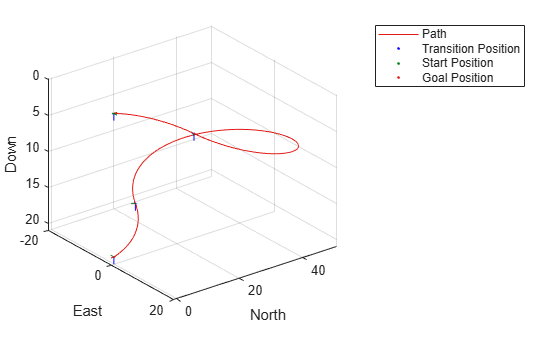# show

Visualize UAV Dubins path segment

## Syntax

``axHandle = show(pathSegObj)``
``axHandle = show(pathSegObj,Name,Value)``

## Description

````axHandle = show(pathSegObj)` plots the path segment with start and goal positions and the transitions between the motion types. NotePlotting uses only the position and the yaw angle. ```

example

````axHandle = show(pathSegObj,Name,Value)` specifies additional name-value pair arguments to control display settings.```

## Examples

collapse all

This example shows how to calculate a UAV Dubins path segment and connect poses using the `uavDubinsConnection` object.

Create a `uavDubinsConnection` object.

`connectionObj = uavDubinsConnection;`

Define start and goal poses as [`x`, `y`, `z`, `headingAngle`] vectors.

```startPose = [0 0 0 0]; % [meters, meters, meters, radians] goalPose = [0 0 20 pi];```

Calculate a valid path segment and connect the poses. Returns a path segment object with the lowest path cost.

`[pathSegObj,pathCosts] = connect(connectionObj,startPose,goalPose);`

Show the generated path.

`show(pathSegObj{1})`Display the motion type and the path cost of the generated path.

`fprintf('Motion Type: %s\nPath Cost: %f\n',strjoin(pathSegObj{1}.MotionTypes),pathCosts);`
```Motion Type: R L R N Path Cost: 138.373157 ```

## Input Arguments

collapse all

Path segment, specified as a `uavDubinsPathSegment` object.

### Name-Value Arguments

Specify optional pairs of arguments as `Name1=Value1,...,NameN=ValueN`, where `Name` is the argument name and `Value` is the corresponding value. Name-value arguments must appear after other arguments, but the order of the pairs does not matter.

Before R2021a, use commas to separate each name and value, and enclose `Name` in quotes.

Example: `'Positions',{'start','goal'}`

Axes used to plot path, specified as the comma-separated pair consisting of `'Parent'` and an `axes` object.

Example: `'Parent',axHandle`

Positions to display, specified as the comma-separated pair consisting of `'Positions'` and a cell array of string or character vectors or a vector of string scalars.

Options are any combination of `'start'`, `'goal'`, and `'transitions'`.

To disable all position displays, specify either as an empty cell array `{}` or empty vector `[]`.

## Output Arguments

collapse all

Axes used to plot path, returned as an `axes` object.

## Version History

Introduced in R2019b